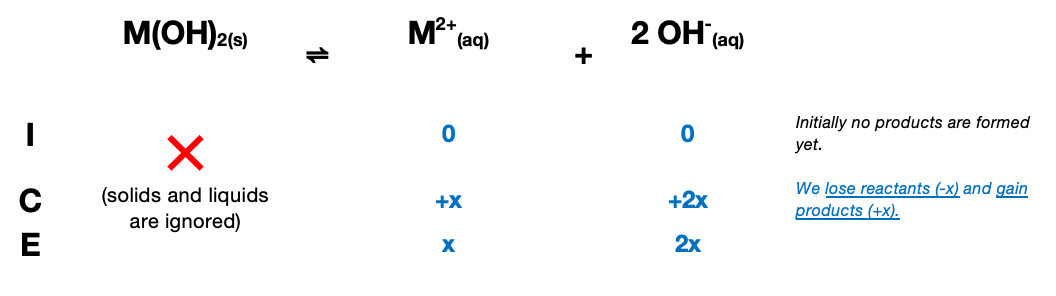# Problem: At 22 °C an excess amount of a generic metal hydroxide M(OH)2 is mixed with pure water. The resulting equilibrium solution has a pH of 10.26. What is the Ksp of the salt at 22 °C?Ksp =

###### FREE Expert Solution

Dissociation Reaction: M(OH)2(s) → M2+(aq) + 2 OH-(aq)$\overline{){{\mathbf{K}}}_{{\mathbf{sp}}}{\mathbf{=}}\frac{\mathbf{product}}{\overline{)\mathbf{reactants}}}{\mathbf{=}}\mathbf{\left[}{\mathbf{M}}^{\mathbf{2}\mathbf{+}}\mathbf{\right]}{\mathbf{\left[}{\mathbf{OH}}^{\mathbf{-}}\mathbf{\right]}}^{{\mathbf{2}}}}$

$\mathbf{pOH}\mathbf{=}\mathbf{14}\mathbf{-}\mathbf{pOH}\mathbf{=}\mathbf{14}\mathbf{-}\mathbf{10}\mathbf{.}\mathbf{26}\mathbf{=}$3.74

$\left[{\mathrm{OH}}^{-}\right]\mathbf{=}{\mathbf{10}}^{\mathbf{-}\mathbf{pOH}}\mathbf{=}{\mathbf{10}}^{\mathbf{-}\mathbf{3}\mathbf{.}\mathbf{74}}\mathbf{=}$1.82×10-4

93% (50 ratings)###### Problem Details

At 22 °C an excess amount of a generic metal hydroxide M(OH)2 is mixed with pure water. The resulting equilibrium solution has a pH of 10.26. What is the Ksp of the salt at 22 °C?

Ksp